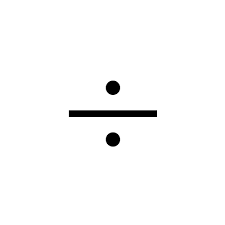# Expressions and Formulas

1.

 (1/2)[32 + (40(-42))]
 a. –824 b. 219 c. –1648 d. 15

2.

 14 – 1805
 a. –22 b. –33.2 c. –4.9 d. –161

3.

 (1/2)[20 + (80(-39))]
 a. –3100 b. –1550 c. 204 d. 30.5

4.

Evaluate the given expression if w = 17, x = 29, y = 48, and z = 8.
w + (1/x) + (1/y) + (1/z)

 a. 17.18 b. 8.11 c. 94.13 d. 46.15

5.

Evaluate the given expression if x = 25, y = 10, w = 11, and z = 18.
(x – y)2 + 10wz

 a. 1995 b. 3205 c. –1755 d. 2205

6.

Find the value of (a/bn) if n = 4, b = 4, and a = 40,000.

 a. 156.25 b. 1562.5 c. 2500 d. 10,000

7.

Find the value of  yn if x = 1000, = 10, and n = 4.

 a.9000 b. 900 c. 990 d. 9000